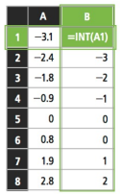Mathematics
Easy

Question

# Results of the INT functions are shown in the spreadsheet.If f(x) = INT(x), what is f(4.6), f(5) and f(-6.5) ?

## 5 , 5 and -6.54.6 , 5 and -6.54, 5 and -75 , 5 and -6Hint:

## The correct answer is: 4, 5 and -7

### Observing the values in the table, we can say that f(x) = floor(x). Floor(4.6) = 4 Floor(5) = 5 Floor(-6.5) = -7

Notice the trend of the function values and identify the function which is used.

### Related Questions to study#### With Turito Foundation.#### Get an Expert Advice From Turito.